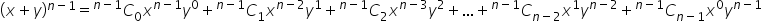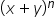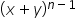Maths-
General
Easy

Question

# How is ( x + y )n obtained from (x + y)n-1Hint:

## The correct answer is: = 1736

### Step 1 of 1:The binomial expansion ofMultiply then expansion by ( x + y ) , that is:You could also get the value offromby just multiplying a (  x + y)  with.#### With Turito Foundation.#### Get an Expert Advice From Turito.